# How to Understand Calculus: Integration Rules and Examples

Eugene is a qualified control/instrumentation engineer Bsc (Eng) and has worked as a developer of electronics & software for SCADA systems.

## How to Understand Calculus

Calculus is a study of rates of change of functions and accumulation of infinitesimally small quantities. It can be broadly divided into two branches:

• Differential Calculus. This concerns rates of changes of quantities and slopes of curves or surfaces in 2D or multidimensional space.
• Integral Calculus. This involves summing infinitesimally small quantities.

## What's Covered in this Tutorial

In this second part of a two part tutorial, we cover:

1. Concept of integration
2. Definition of indefinite and definite integrals
3. Integrals of common functions
4. Rules of integrals and worked examples
5. Applications of integral calculus, volumes of solids, real world examples

## Integration is a Summing Process

We saw in the first part of this tutorial how differentiation is a way of working out the rate of change of functions. Integration in a sense is the opposite of that process. It is a summing process used to add up infinitesimally small quantities.

## What is Integral Calculus Used For?

Integration is a summing process, and as a mathematical tool it can be used for:

• evaluating the area under functions of one variable
• working out the area and volume under functions of two variables or summing multidimensional functions
• calculating the surface area and volume of 3D solids

In science, engineering, economics etc, real world quantities such as temperature, pressure, magnetic field strength, illumination, speed, flow rate, share values etc can be described by mathematical functions. Integration allows us to integrate these variables to arrive at a cumulative result.

## Area Under a Graph of a Constant Function

Imagine we have a graph showing the velocity of a car versus time. The car travels at a constant velocity of 50 mph, so the plot is just a horizontal straight line.

The equation for distance travelled is:

Distance travelled = velocity x time

So in order to calculate distance travelled at any point in the journey, we multiply the height of the graph (the velocity) by the width (time) and this is just the rectangular area under the graph of velocity. We are integrating velocity to calculate distance. The resulting graph we produce for distance versus time is a straight line.

Scroll to Continue

So if the car's velocity is 50 mph, then it travels

50 miles after 1 hour

100 miles after 2 hours

150 miles after 3 hours

200 miles after 4 hours and so on.

Note that an interval of 1 hour is arbitrary, we can choose it to be anything we want.

If we draw a graph of distance travelled versus time, we see how the distance increases with time. The graph is a straight line.

## Area Under a Graph of a Linear Function

Now let's make things a little bit more complicated!
This time we'll use the example of filling a water tank from a pipe.

Initially there is no water in the tank and no flow into it, but over a period of minutes, the flow rate increases continuously.

The increase in flow is linear which means that the relationship between flow rate in gallons per minute and time is a straight line.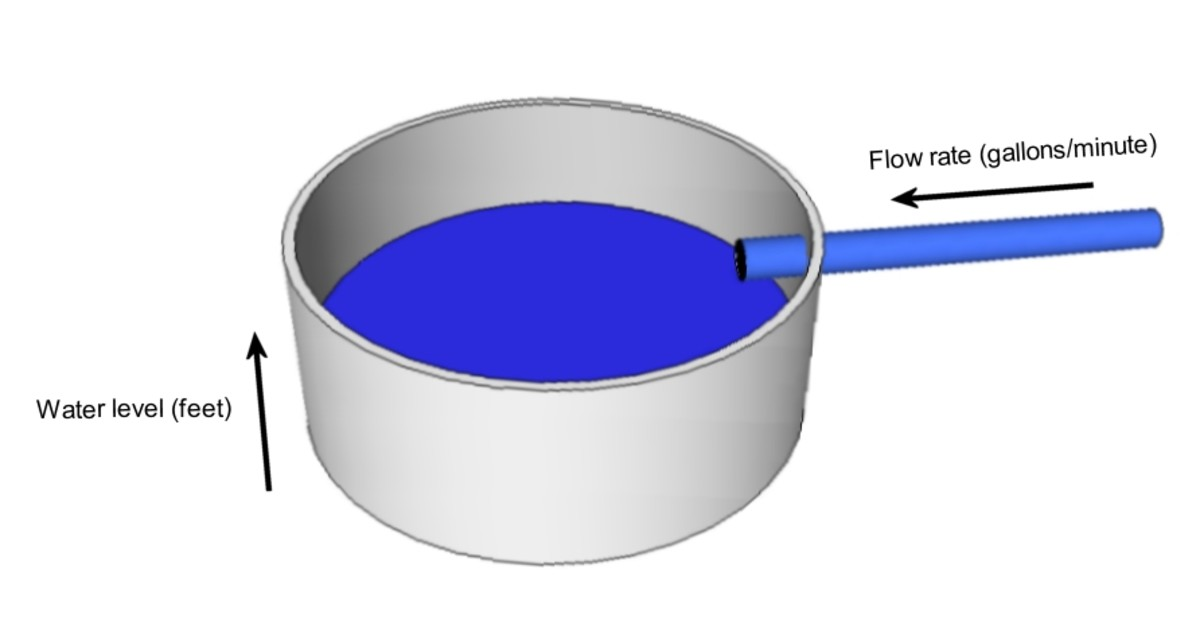A tank filling with water. Water volume increases and is the integral of flow rate into the tank.© Eugene Brennan

We use a stopwatch to check elapsed time and record the flow rate every minute. (Again this is arbitrary).

After 1 minute, flow has increased to 5 gallons per minute.

After 2 minutes, flow has increased to 10 gallons per minute.

and so on.....

Flow rate is in gallons per minute (gpm) and volume in the tank is in gallons.

The equation for volume is simply:

Volume = average flow rate x time

Unlike the example of the car, to work out the volume in the tank after 3 minutes, we can't just multiply the flow rate (15 gpm) by 3 minutes because the rate was not at this rate for the full 3 minutes. Instead we multiply by the average flow rate which is 15/2 = 7.5 gpm.

So volume = average flow rate x time = (15/2) x 3 = 2.5 gallons

In the graph below, this just turns out to be the area of the triangle ABC.

Just like the car example, we are calculating the area under the graph.

If we record the flow rate at intervals of 1 minute and work out the volume, the increase in water volume in the tank is an exponential curve.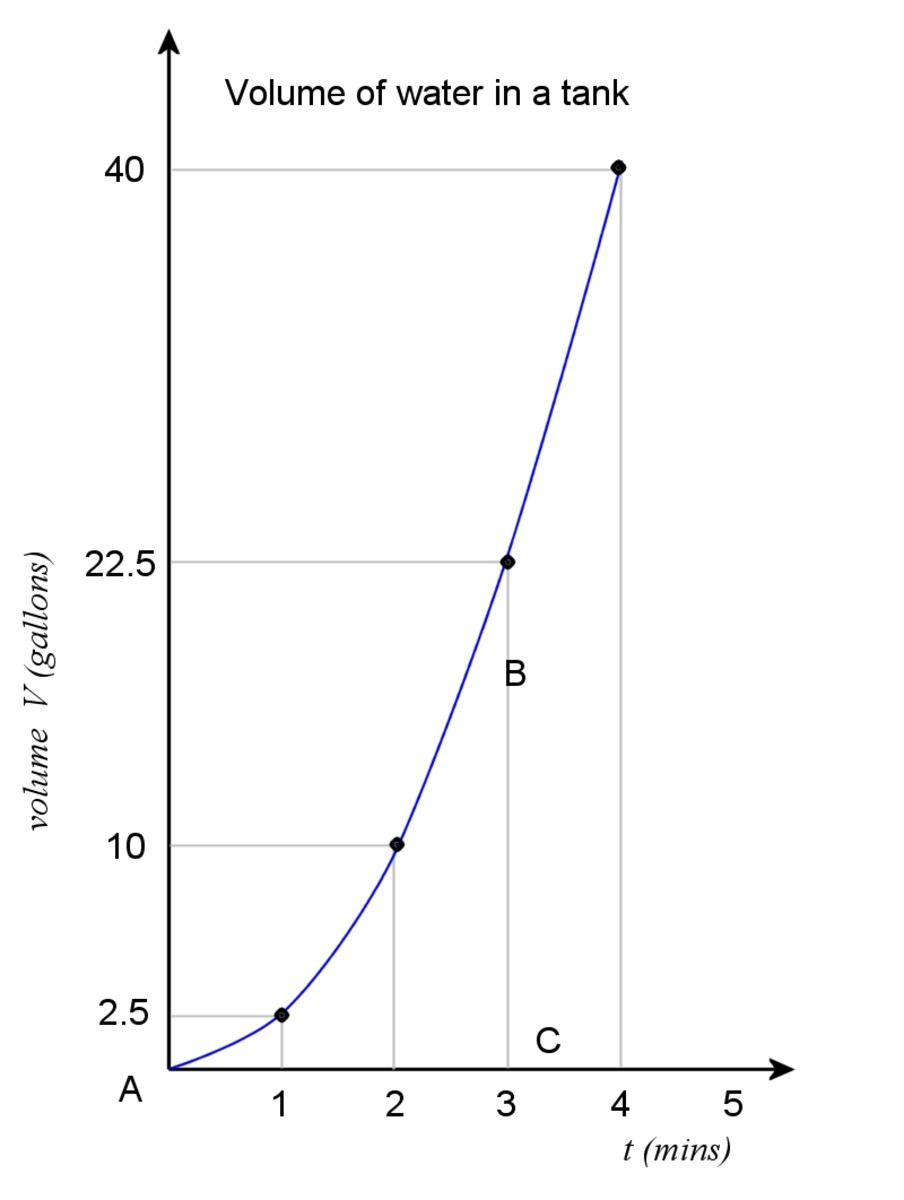Plot of water volume. Volume is the integral of flow rate into the tank.© Eugene Brennan

Now consider a case where the flow rate into the tank is variable and non-linear. Again we measure the flow rate at regular intervals. Just like before, the volume of water is the area under the curve. We can't use a single rectangle or triangle to calculate area, but we can try to estimate it by dividing it up into rectangles of width Δt, calculating the area of those and summing the result. However there will be errors and the area will be underestimated or over estimated depending on whether the graph is increasing or decreasing.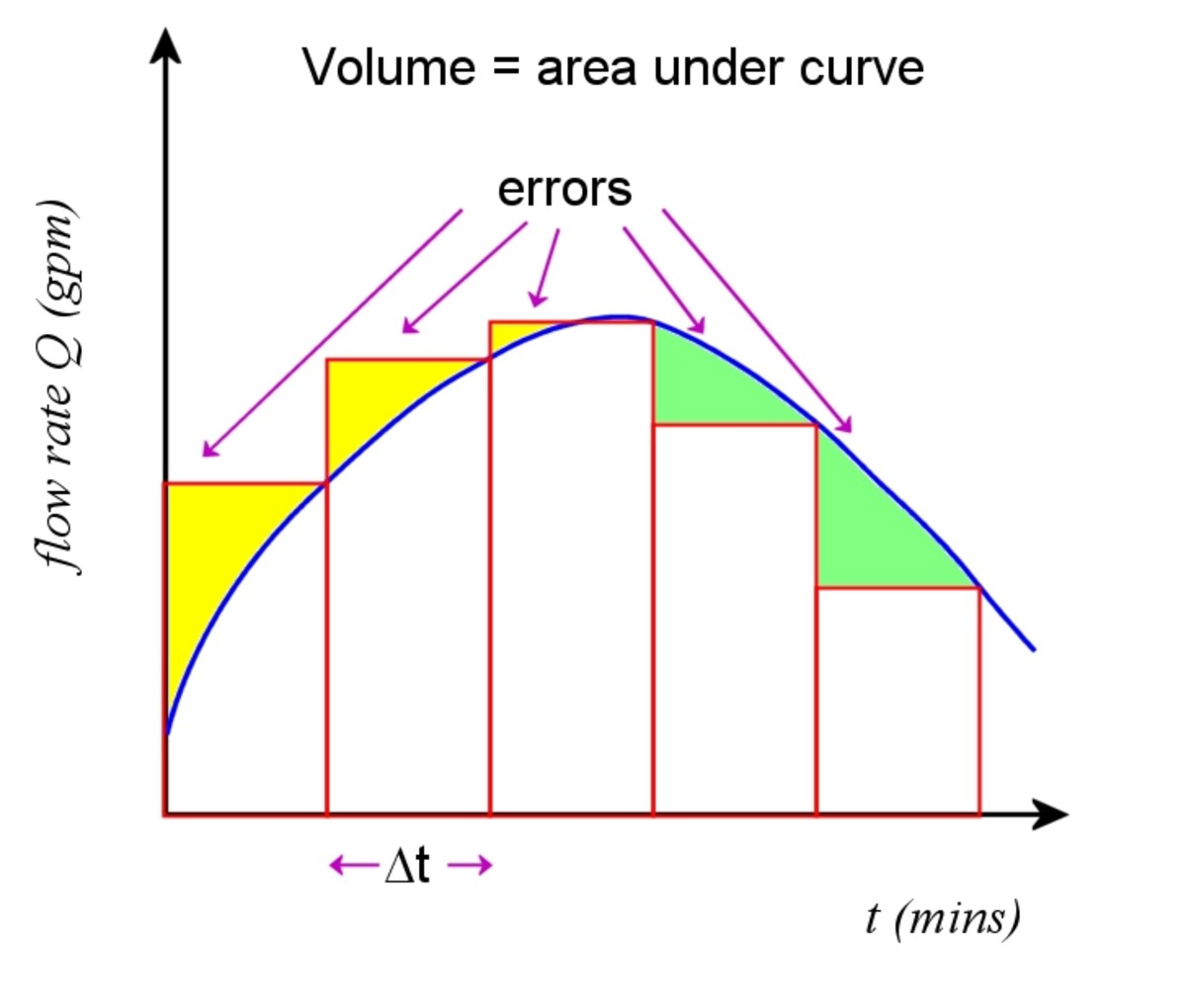We can get an estimate of area under the curve by summing a series of rectangles.© Eugene Brennan

## Using Numerical Integration to Find the Area Under a Curve.

We can improve accuracy by making the intervals Δt shorter and shorter.

We are in effect using a form of numerical integration to estimate the area under the curve by adding together the area of a series of rectangles.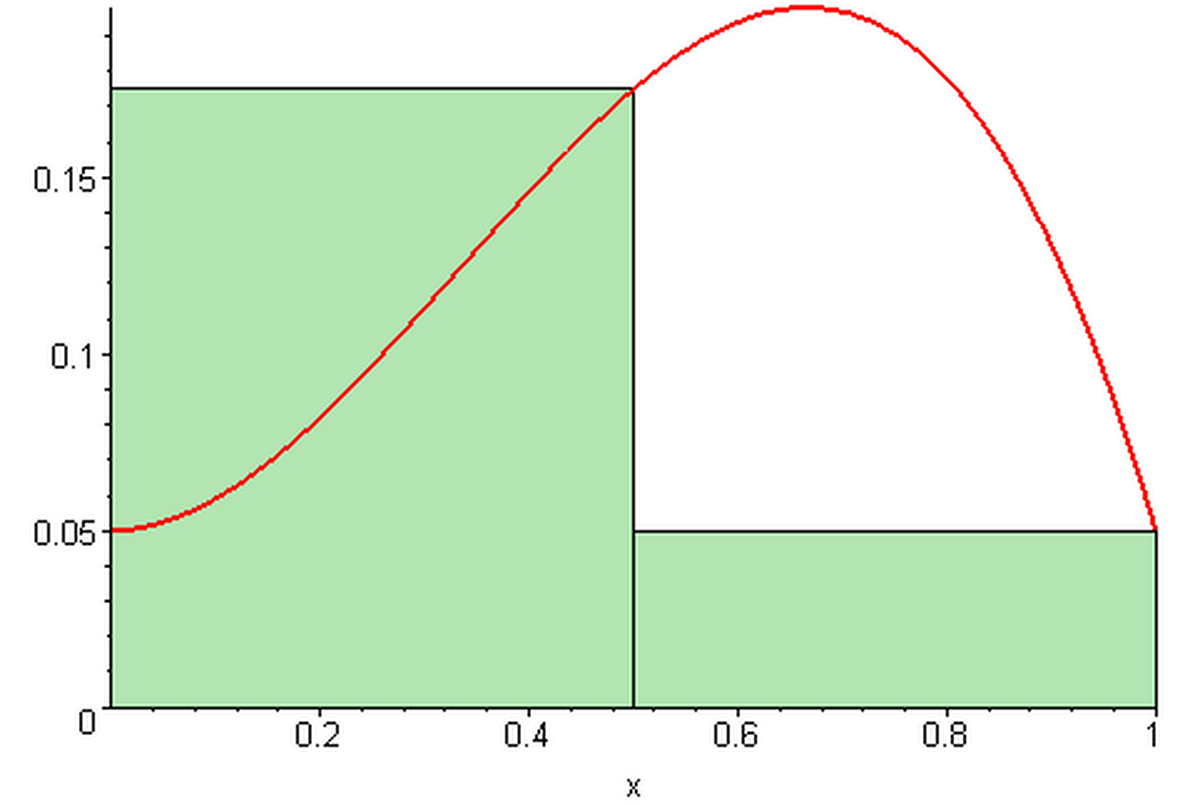As the number of rectangles gets bigger and their width gets smaller, the errors get smaller and the result more closely approximates the area under the curve.09glasgow09, CC BY SA 3.0 via Wikimedia Commons

Now consider a general function y = f (x).

We are going to specify an expression for the total area under the curve over a domain [a,b] by summing a series of rectangles. In the limit, the width of the rectangles will become infinitesimally small and approach 0. The errors will also become 0.

• The result is called the definite integral of f (x) over the domain [a,b].
• The ∫ symbol means "the integral of" and the function f (x) is being integrated.
• f (x) is called an integrand.

The sum is called a Riemann Sum. The one we use below is called a right Reimann sum. dx is an infinitesimally small width. Roughly speaking, this can be thought of as the value Δx becomes as it approaches 0. The Σ symbol means that all the products f (xi)xi (the area of each rectangle) are being summed from i = 1 to i = n and as Δx → 0, n → ∞.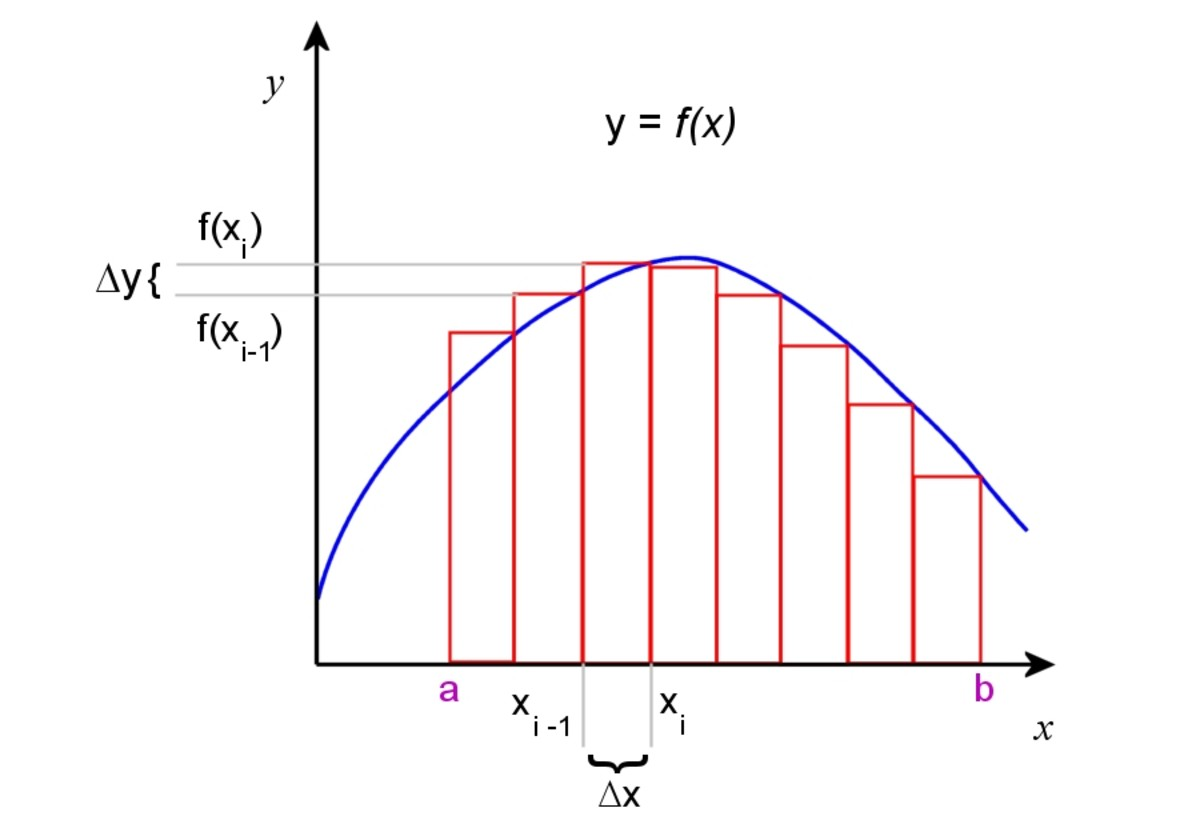A generalised function f(x). Rectangles can be used to approximate the area under the curve.© Eugene Brennan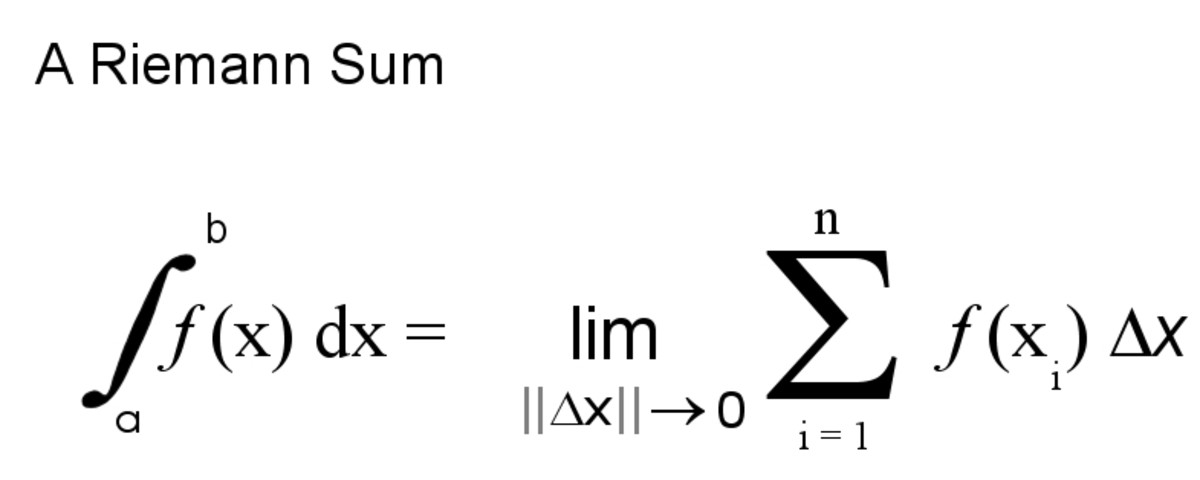Right Riemann sum. In the limit as Δx approaches 0, the sum becomes the definite integral of f(x) over the domain[a,b].© Eugene Brennan

## The Difference Between Definite and Indefinite Integrals

Analytically we can find the anti-derivative or indefinite integral of a function f (x).
This function has no limits.
If we specify an upper and lower limit, the integral is called a definite integral.

## Using Indefinite Integrals to Evaluate Definite Integrals

If we have a set of data points, we can use numerical integration as described above to work out the area under curves. Although it wasn't called integration, this process has been used for thousands of years to calculate area and computers have made it easier to do the arithmetic when thousands of data points are involved.
However if we know the function f (x) in equation form (e.g. f (x) = 5x2 + 6x +2) , then firstly knowing the anti-derivative (also called the indefinite integral) of common functions and also using rules of integration, we can analytically work out an expression for the indefinite integral.

The fundamental theorem of calculus then tells us that we can work out the definite integral of a function f(x) over an interval using one of its anti-derivatives F(x). Later we will discover that there are an infinite number of anti-derivatives of a function f (x).

## Indefinite Integrals and Constants of Integration

The table below shows some common functions and their indefinite integrals or anti-derivatives. C is a constant. There are an infinite number of indefinite integrals for each function because C can have any value.

Why is this?

Consider the function f (x) = x3

We know the derivative of this is 3x2

What about x3 + 5 ?

d/dx ( x3 + 5 ) = d/dx (x3) + d/dx(5) = 3x2 + 0 = 3x2 ....... the derivative of a constant is 0

So the derivative of x3 is the same as the derivative of x3 + 5 and = 3x2

What's the derivative of x3 + 3.2 ?

Again d/dx ( x3 + 3.2 ) = d/dx (x3) + d/dx(3.2) = 3x2 + 0 = 3x2

No matter what constant is added to x3, the derivative is the same.

Graphically we can see that if functions have a constant added, they are vertical translations of each other, so since the derivative is the slope of a function, this works out the same no matter what constant is added.

Since integration is the opposite of differentiation, when we integrate a function, we must add on a constant of integration to the indefinite integral

So e.g. d/dx( x3) = 3x2

and ∫ 3x2 dx = x3 + C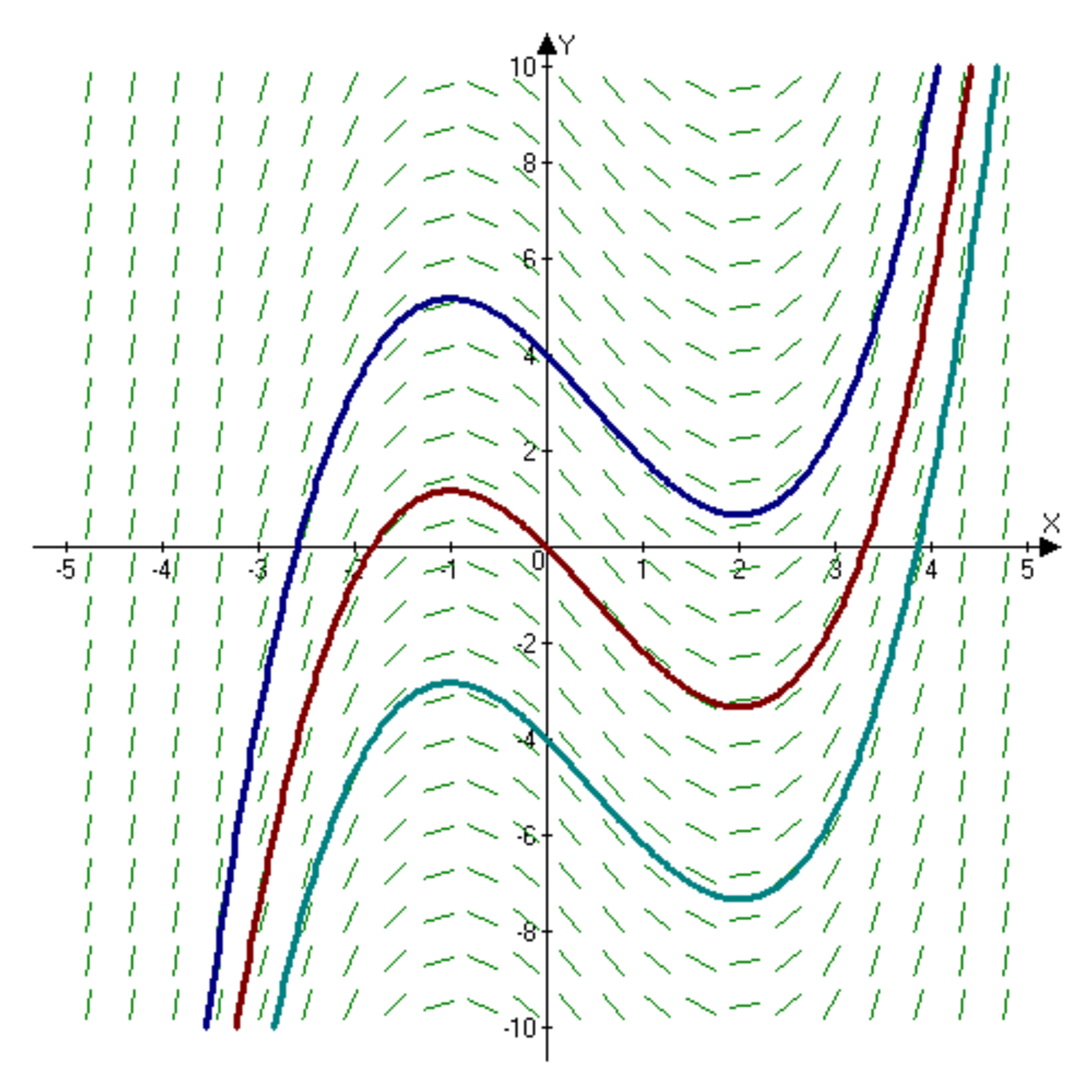Slope field of a function x^3/3 - x^2/2 - x + c, showing three of the infinite number of functions that can be produced by varying the constant c. The derivative of all the functions is the same.pbroks13talk, public domain image via Wikimedia Commons

## Indefinite Integrals of Common Functions

Function TypeFunctionIndefinite Integral

Constant

∫ a dx

ax + C

Variable

∫ x dx

x² / 2 + C

Reciprocal

∫ 1/x dx

ln x + C

Square

∫ x² dx

x³ / 3 + C

Trigonometric Functions

∫ sin (x) dx

- cos (x) + C

∫ cos (x) dx

sin (x) + C

∫ sec ² (x) dx

tan (x) + C

Exponential functions

∫ eˣ dx

eˣ + C

∫ aˣ dx

(aˣ) / ln (a) + C

∫ ln (x) dx

xln (x) - x + C

In the table below, u and v are functions of x.

u ' is the derivative of u wrt x.

v ' is the derivative of v wrt x.

## Rules of Integration

RuleFunctionIntegral

Multiplication by a constant rule

∫ au dx

a ∫ u dx

Sum rule

∫ ( u + v ) dx

∫ u dx + ∫ v dx

Difference rule

∫ ( u - v ) dx

∫ u dx - ∫ v dx

Power rule (n ≠ -1)

∫ (xⁿ) dx

x ⁽ⁿ ⁺ ¹⁾ / (n + 1) + C

Reverse chain rule or integration by substitution

∫ f(u) u ' dx

∫ f(u) du + C ................... Replace u'(x)dx by du and integrate wrt u, then substitute back for the value of u in terms of x in the evaluated integral.

Integration by parts

∫ u v ' dx

u v - ∫ u ' v dx

## Examples of Working Out Integrals

Example 1:

Evaluate ∫ 7 dx

∫ 7 dx =

7 ∫ dx ..........multiplication by a constant rule

= 7x + C

Example 2:

What is ∫ 5x4 dx

5x4 dx = 5 x4 dx ....... using multiplication by a constant rule

= 5(x5/5) + C .......... using power rule

= x5 + C

Example 3:

Evaluate ∫ (2x3 + 6cos(x) ) dx

∫ (2x3 + 6cos(x) ) dx = ∫ 2x3 dx + ∫ 6cos(x) dx .....using the sum rule

= 2 ∫ x3 dx + 6 ∫ cos(x) dx ..........using the multiplication by a constant rule

= 2(x4/4) + C1 + 6(sin(x) + C2 .....using the power rule. C1 and C2 are constants.

C1 and C2 can be replaced by a single constant C, so:

∫ (2x3 + cos(x) ) dx = x4/2 + 6sin(x) + C

Example 4:

Work out ∫ sin2(x)cos(x) dx

• We can do this using the reverse chain rule ∫ f(u) u'(x) dx = ∫ f(u) du where u is a function of x
• We use this when we have an integral of a product of a function of a function and its derivative

sin2(x) = (sin x) 2

Our function of x is sin x so replace sin(x) by u giving us:

u = sin(x)

u' = cos(x)

sin2(x) = f(u) = u2

Replace cos(x)dx by du

So ∫ sin2(x)cos(x) dx = ∫ u2du = u3/3 + C

Substitute u = sin(x) back into the result:

u3/3 + C = sin3(x)/3 + c

So ∫ sin2(x)cos(x) dx = sin3(x)/3 + c

Example 5:

Evaluate ∫ xex^2 dx

It looks as though we could use the reverse chain rule for this example because 2x is the derivative of the exponent of e which is x2. However we need to adjust the form of the integral first.
So write ∫ xex^2 dx as 1/2 x ∫ 2xex^2 dx = 1/2 ∫ ex^2 (2x) dx

No we have the integral in the form ∫ f(u) u' dx where u = x2 and du = 2xdx

Substituting for x2 and 2x dx:

1/2 ∫ ex^2 (2x) dx = 1/2 ∫ eu u' dx = 1/2 ∫ eu du

but the integral of the exponential function eu is itself, so:

1/2 ∫ eu du = 1/2 eu

Substitute for u giving

1/2 eu = 1/2 ex^2

Example 6:

Evaluate ∫ 6/(5x + 3) dx

• For this, we can use the reverse chain rule again.
• We know that 5 is the derivative of 5x + 3.

Rewrite the integral so that 5 is within the integral symbol and in a format that we can use the reverse chain rule:

∫ 6/(5x + 3) dx = ∫ (6/5) 5/(5x + 3) dx = 6/5 ∫ 1/(5x + 3) 5 dx

Again we have our reverse chain rule ∫ f(u) u' dx = ∫ f(u) du

Let u = 5x + 3

u' = 5 and du = 5dx

Plug these into the integral:

6/5∫ 1/(5x + 3)5dx = 6/5∫ (1/u) du

But ∫ (1/u) du = ln(u) + C

So substituting back 5x + 3 for u gives:

∫ 6/(5x + 3) dx = 6/5∫ (1/u) du = 6/5ln(u) + C = 1.2ln(5x + 3) + C

Stroud, K.A., (1970) Engineering Mathematics (3rd ed., 1987) Macmillan Education Ltd., London, England.

Eugene Brennan (author) from Ireland on June 21, 2019:

Hi Patty,

Thanks for having a look at this and for the comments!

The green image isn't mine, but I have a similar animated GIF on the companion article about differentiation. It's possible to do really fancy graphs using the online/downloadable GeoGebra application, but unfortunately the graphs can't be used free for commercial purposes and a licence needs to be bought. I might try to negotiate with them.

Have a great weekend yourself!

Eugene

Patty Inglish MS from USA and Asgardia, the First Space Nation on June 21, 2019:

Hi Eugene -

I like the shade of light green you use in the graphs that feature color and especially like the image that both moves and includes the green shading.

Calculus was interesting when I studied it, but I would have enjoyed the colors and your images - they are clearer than those we had in our books.

Have a good weekend!